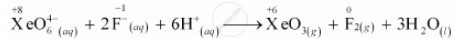CBSE (Science) Class 11CBSE
Share

# Why Does the Following Reaction Occur?What Conclusion About the Compound Na4xeo6 (Of Which Xeo_6^(4+) is a Part) Can Be Drawn from the Reaction. - CBSE (Science) Class 11 - Chemistry

ConceptOxidation Number Types of Redox Reactions

#### Question

Why does the following reaction occur?

XeO_6^(4-) (aq) + 2F^(-) (aq) + 6H^(+) (aq) -> XeO_3(g) + F_2(g) + 3H_2O(l)

What conclusion about the compound Na4XeO6 (of which XeO_6^(4+) is a part) can be drawn from the reaction.

#### Solution 1Here O.N of Xe decreases from +8 in XeO_6^(4-) to +6 in XeO_3 while that of F increase from-I in F^(-) to 0 in F_2. Therefore XeO_6^(4-) is reduced while F^(-) is oxidised. This reaction occur because Na_2XeO_6^(4-) (or XeO_6^(4-)) is stronger oxidising  agent than F_2

#### Solution 2

The given reaction occurs because XeO_6^(4-) oxidises F^(-) and F^(-) reduces XeO_6^(4-)In this reaction, the oxidation number (O.N.) of Xe decreases from +8 in XeO_6^(4-)

to +6 in XeO3 and the O.N. of F increases from –1 in F– to O in F2.

Hence, we can conclude that Na_4XeO_6 is a stronger oxidising agent than F.

Is there an error in this question or solution?

#### APPEARS IN

Solution Why Does the Following Reaction Occur?What Conclusion About the Compound Na4xeo6 (Of Which Xeo_6^(4+) is a Part) Can Be Drawn from the Reaction. Concept: Oxidation Number - Types of Redox Reactions.
S